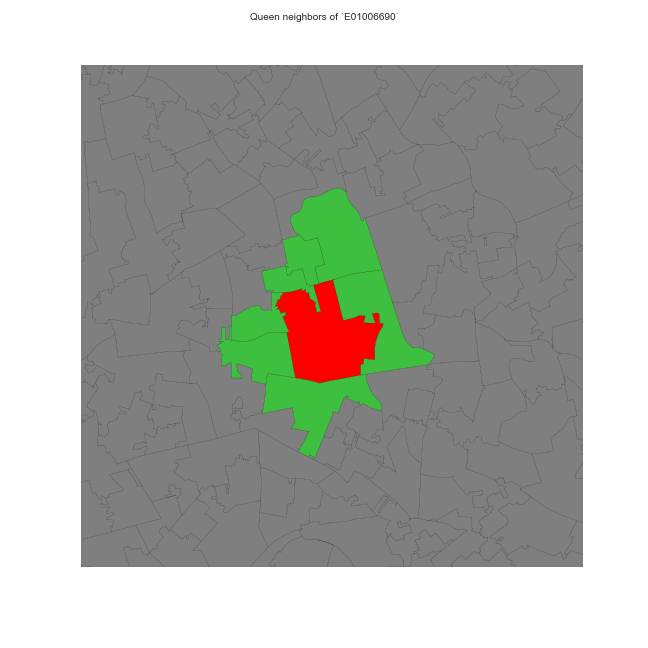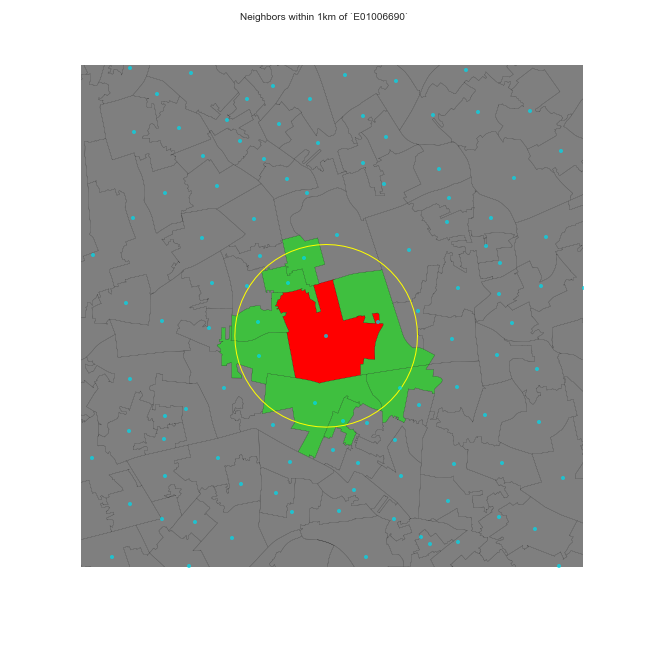Types of W
Dani Arribas-Bel

## What is a neighbor?

A neighbor is “somebody” who is:

• Next door Contiguity-based Ws
• Close Distance-based Ws
• In the same “place” as us Block weights

See Anselin & Rey (2014) for an in-detail discussion and more types of W.

## Contiguity-based weights

Sharing boundaries to any extent

• Rook
• Queen

## Distance-based weights

Weight is (inversely) proportional to distance between observations

• Inverse distance (threshold)
• KNN (fixed number of neighbors)## Block weights

Weights are assigned based on discretionary rules loosely related to geography

For example:

• LSOAs into MSOAs
• Post-codes within city boundaries
• Counties within states

## How much of a neighbor?

No neighbors receive zero weight: wij = 0

Neighbors, it depends, wij can be:

• One wij = 1 Binary

• Some proportion (0 < wij < 1, continuous) which can be a function of:

• Distance
• Strength of interaction (e.g. commuting flows, trade, etc.)

## Choice of W

Should be based on and reflect the underlying channels of interaction for the question at hand.

Examples:

• Processes propagated by inmediate contact (e.g. disease contagion) Contiguity weights
• Accessibility Distance weights
• Effects of county differences in laws Block weights

## Standardization

In some applications (e.g. spatial autocorrelation) it is common to standardize W

The most widely used standardization is row-based: divide every element by the sum of the row:

where wi· is the sum of a row.